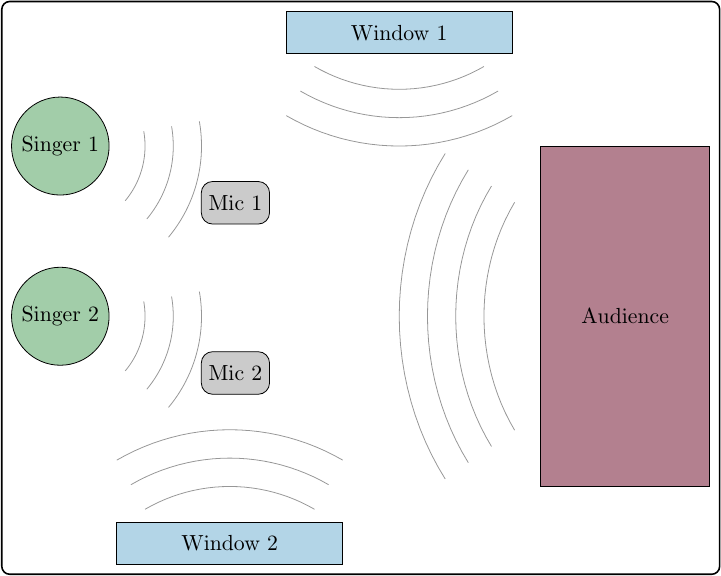# coroICA

This site accompanies the following JMLR paper on an extension to the well-known independent component analysis (ICA) for blind source separation. The extension demixes signals that are generated as follows:

$$X_i = A \cdot S_i + H_i$$ where
• $S_i = (S_i^1, ...., S_i^d)^\top \in \mathbb{R}^d$ and $H_i = (H_i^1, ..., H_i^d)^T \in \mathbb{R}^d$ are two independent sequences of random vectors,
• the components $S_i^1, ..., S_i^d$ are mutually independent for all $i$,
• $A \in \mathbb{R}^{d\times d}$ is an invertible mixing matrix,
• the confounding terms $H_i$ have fixed covariance within groups, i.e., $$\operatorname{Cov}(H_i)=\operatorname{Cov}(H_j)$$ where $i$, $j$ are observations from the same group.
Python implementation
An open-source scikit-learn compatible Python implementation of this algorithm can be installed from PyPI. The Python source is available on github; please report issues there or fire a pull request if you wish to contribute. Please refer to the Python documentation, getting started in Python, as well as the minimalistic example to get started.
The experimental results presented in the manuscript can be reproduced using this code archive.
R implementation
An open-source R implementation of this algorithm can be installed from CRAN. The R source is available on github; please report issues there or fire a pull request if you wish to contribute. The documentation ships with the CRAN package.
Matlab implementation
An open-source Matlab implementation of this algorithm can be installed from the Matlab source available on github; please report issues there or fire a pull request if you wish to contribute. A basic docstring is provided to get you started.

## Contents

### "America's Got Talent Duet Problem" -- an audible example

Consider we are recording from two microphones in a setting as it is sketched below, where two singers perform a duet on stage while we may want to judge the individual's performance.#### Original signal recorded at microphones (real scrambled)

Let's first see how the signals recorded in such a setting may sound like.
Signal Audience noise Open window (birds) Mower Applause
1

2

The signals are mixed to an extent where it is sometimes hard to follow one of the two speeches, which are interwovenly recorded at both microphones.

#### Signals recovered by pooled ICA (still scrambled)

As a first classical attempt we may apply ICA in order to do blind source separation and hopefully recover the two speeches from the microphone recordings. Here is what pooled ICA can do for us:
Signal Audience noise Open window (birds) Mower Applause
1

2

#### Signals recovered by coroICA (much better)

Since the data has a grouped structure, e.g., there is periods where someone opened the window and we can hear a clear mower sound etc., it falls into the regime of the coroICA model. Applying coroICA that properly accounts for this grouped structure we are able to recover the two speeches as follows:
Signal Audience noise Open window (birds) Mower Applause
1

2

### EEG Data -- an example comparing to pooledICA

A common application of ICA is in the analysis of EEG (Electroencephalography) data. To illustrate a potential use of coroICA, we apply it to the publicly available multi-subject data set Covert shifts of attention, which is preprocessed as described in our manuscript (Data Set 3).

In this illustration, we select one subject and learn an unmixing matrix with coroICA, pooledICA and a random projection on the remaining 7 subjects. For each of the 3 unmixing matrices, we then construct the following two types of topographic maps on the left-out subject:

1. A topographic map of $a_j$, where $a_j$ is the $j$-th column of the mixing matrix. This topographic map illustrates the mixing of the $j$-th source at each electrode position.
2. A topographic map of $\operatorname{cov}(\mathbf{X_t})v_j^\top$, where $v_j$ is the $j$-th row of the unmixing matrix and $\operatorname{cov}(\mathbf{X_t})$ is the covariance matrix of the observed data at time $t$ estimated with a moving-window estimator. This topographic map illustrates the time-dependent source activation of the recovered $j$-th source at each electrode.

The resulting plots are illustrated in the following video. Here, the first row corresponds to the topographic map (1) and the second row to the one described in (2).

Given an underlying ICA model and a good estimation the time changing topographic maps in the second row should correspond to the first row. In this particular example, one can see that the source recovered by coroICA remains more stable across time and is able to capture the overall structure more consistently. See also our manuscript for more details on this.

### Getting started in Python

We have made our code available as a scikit-learn compatible package. The coroICA package can be installed from PyPI using the following command:
pip install coroICA

The default usage requires a data matrix $X\in\mathbb{R}^{n\times d}$, an array $\operatorname{groups}\in\mathbb{R}^{n}$ specifing to which group each of the $n$ observations belongs and a second array $\operatorname{partition}\in\mathbb{R}^{d}$ specifing the partition. The sources can then be recovered using the following commands:
from coroica import CoroICA

c = CoroICA()
c.fit(Xtrain, group_index=groups, partition_index=partition)
# c.V_ holds the unmixing matrix

recovered_sources = c.transform(Xtest)

Using our package, one can also run other second-order-statistics-based ICA algorithms as mentioned in our paper, the transformers are instantiated as follows:
from coroica import UwedgeICA

SOBI = UwedgeICA(partitionsize=int(10**6), timelags=list(range(1, 101)))

choiICA_var = UwedgeICA()

choiICA_var_TD = UwedgeICA(timelags=[1, 2, 3, 4, 5])

choiICA_TD = UwedgeICA(instantcov=False, timelags=[1, 2, 3, 4, 5])

For a more in-depth example and further details on the package, see the documentation.
The presented educational audible example combines parts of these sounds from freesound "Crowd ambience, eating popcorn.wav" by IllusiaProductions (https://freesound.org/people/IllusiaProductions/sounds/249940/), "Ambience, Food Court, B.wav" by InspectorJ (https://freesound.org/people/InspectorJ/sounds/421715/), "rbh Applause 01 big.WAV" by RHumphries (https://freesound.org/people/RHumphries/sounds/1921/), "Birds awaking" by arnaud coutancier (https://freesound.org/people/arnaud%20coutancier/sounds/427335/), "lawn mower (from in house)" by rayjensen (https://freesound.org/people/rayjensen/sounds/347227/); as well as parts of the audio of the Final Presidential State of the Union Address 2016 (http://www.americanrhetoric.com/speeches/stateoftheunion2016.htm) and 2018 (http://www.americanrhetoric.com/speeches/stateoftheunion2018.htm) which are in the public domain or property of AmericanRhetoric.com (http://www.americanrhetoric.com/copyrightinformation.htm).